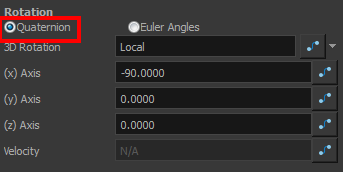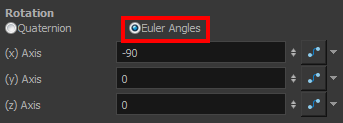# About the 3D Rotation Parameter

The main parameter you will use when working in three-dimensions is rotation. Without using 3D rotation, you are simply working using the multiplane technique.3D rotation can be used in two different ways:

##### Quaternion RotationQuaternion Rotation is very similar to the 3D path position parameters. A Quaternion is composed of three rotation functions: X, Y and Z to control the rotation on three axes, and a velocity function to control the velocity of the three rotation functions simultaneously.

The X, Y and Z functions are linked. When you add a keyframe on one of them, it also adds it to the two others.

If you are planning to animate an element such as the camera, you should use the Quaternion rotation rather than Euler Angles. Quaternion creates a smooth trajectory and interpolation since the path is controlled by the same keyframes and a single velocity.

## Euler Angles RotationThe Euler Angles Rotation is very similar to the Separate position parameters. The Euler Angles parameter is composed of three functions: X, Y and Z to control the rotation on three axes. These three functions are independent of each other; each has its own velocity.

When you add a keyframe on one function, the other ones do not receive it, allowing you to control each axis independently.

Euler Angles are mainly used to position elements with exact coordinates and rotate elements on a single axis. The interpolation between the keyframes will not be as smooth as the Quaternion one since each function works individually.